# Getting started with PyRETIS¶

After installing PyRETIS, you can run simulations by using a text based input file or by using the PyRETIS library explicitly.

The former approach is perhaps the simplest, but you will first have to learn how to create input files and this is explained in the input description.

The latter option is more involved, but you are then given more freedom in defining, running and interacting with a simulation. In order to make use of the library, you will have to read about the structure of the PyRETIS library in the introduction to the library and in the detailed reference section.

A more extensive overview can be found in the full user guide. Here, we will now rather jump into some examples showing the use of PyRETIS.

## PyRETIS examples¶

In addition to reading the documentation, we have prepared several examples to highlight the usage and capabilities of PyRETIS. The full list of examples can be found in the example section.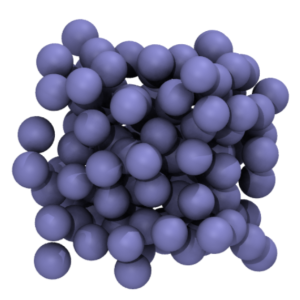Molecular dynamics

In this example, we simply run a MD simulation. This is just intended as an example of how one can make use of PyRETIS as a library.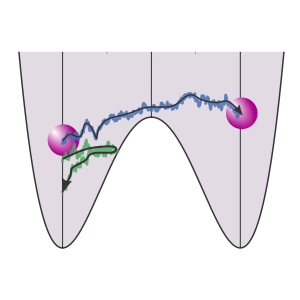TIS

This example shows how we can run a Transition Interface Sampling calculation and obtain a crossing probability. Here, we consider a 1D potential in which a single particle is moving.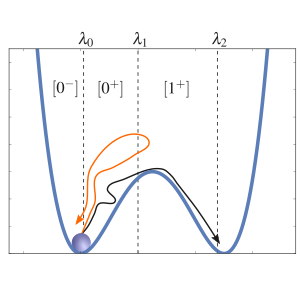RETIS

This example show how we can run a Replica Exchange Transition Interface Sampling calculation and obtain a crossing probability and a rate constant. Here, we consider a 1D potential in which a single particle is moving.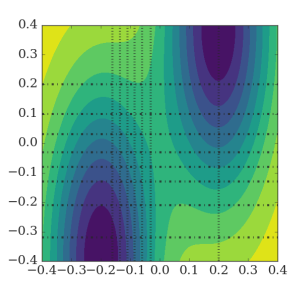RETIS 2D

In this example, we perform a simulation of a 2D potential which is constructed such that the selection of the order parameter is not so obvious.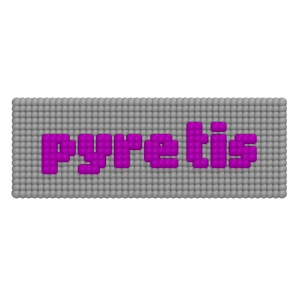Extending with C/FORTRAN

This example shows how we can use FORTRAN or C to speed up PyRETIS calculations.Using OpenMM

This example demonstrates how we interface between OpenMM and PyRETIS internal code.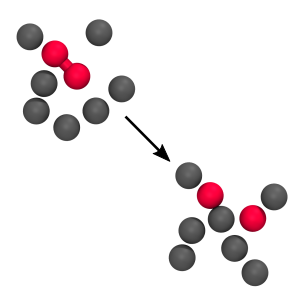RETIS 2D WCA

Here we calculate the rate for the breaking of a bond between two particles in a fluid. We consider two cases: a low barrier and a high barrier case and we implement the force field in C.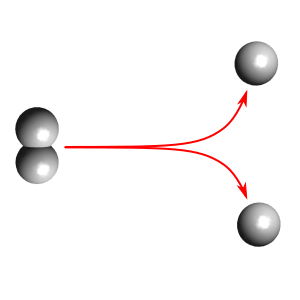Using CP2K

This example demonstrates how we can make use of CP2K for running the dynamics for PyRETIS. Here, we are just studying a toy example - breakage of the bond in hydrogen.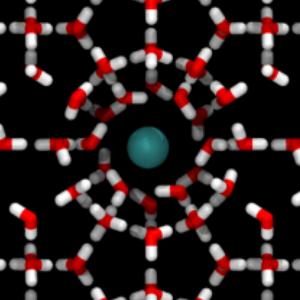Using GROMACS

This example demonstrates how we can make use of GROMACS for running the dynamics for PyRETIS. In this particular example, we study the diffusion of methane in a sI hydrate structure.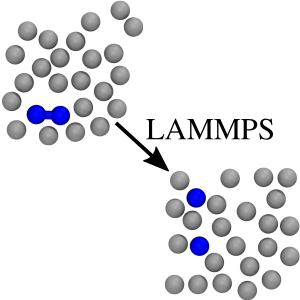Using LAMMPS

This example demonstrates how we can make use of LAMMPS for running the dynamics for PyRETIS. In this particular example, we revisit the 2D WCA example and use LAMMPS as our molecular dynamics engine.

## Main studies performed by PyRETIS¶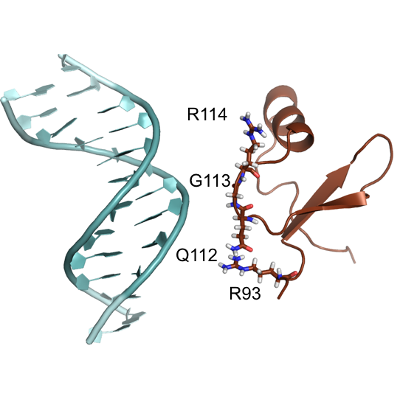Predicting the mechanism and rate of H-NS binding to AT-rich DNA.

The adsorption of H-NS on DNA has been studied at full atomistic resolution with the GROMACS simulation package. Local minima have been located by metadynamics and the relative transition rates computed by RETIS. More information can be found in the paper Predicting the mechanism and rate of H-NS binding to AT-rich DNA.

‘Source Files: <http://pyretis.org/source_files/H-NS_2019>’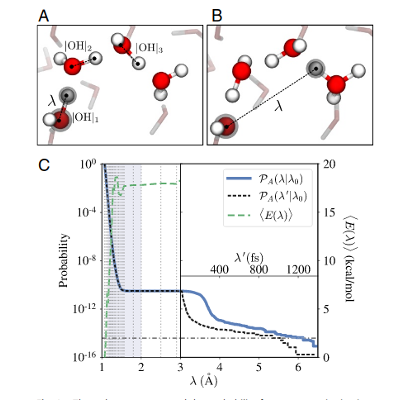Autoionization of water.

BO-DFT simulations, via the RETIS approach, were used to study water autoionization. The mechanism(s) have been highlighted and their rate(s) quantified. Machine learning was applied to test the quality of the order parameters. More information can be found in the paper Local initiation conditions for water autoionization.

‘Source Files: <http://pyretis.org/source_files/Water_2019>’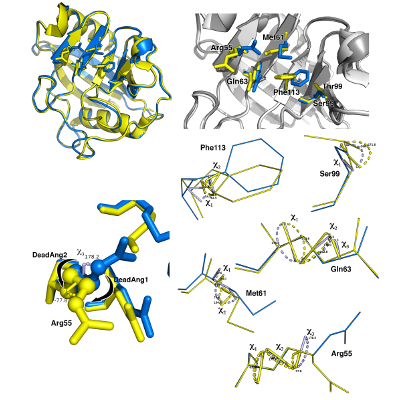Conformational study of Cyclophilin - A.

Full atomistic simulations with GROMACS have been performed to sample and quantify the rate of the structural rearrangements of CyP-A and its muted confomer.

Article in preparation. ‘Source Files: <http://pyretis.org/source_files/CyP-A_2019>’